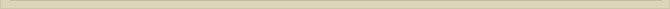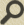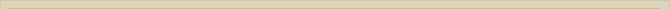## The ether again

When you are interested in physics you must read “Unbelievable“!

For the ether theory the ether itself is of course of the utmost importance. A description how the ether contributes to physical processes is inevitable. The examination given in the previous chapters is not scientific but nevertheless necessary to be able to understand the underlying processes. There is no other way to describe the physical phenomena. This problem does not occur with the present perception of Theoretical Physics because the vacuum is assumed relative and absolutely empty. A description of the physical processes that occur is hardly possible when the physical background is determined the relativity of time and space. The mathematical interpretation determines the physical outcome completely.

With the ether theory this is not the case. The description of the processes taking place in the ether is the most essential part. The mathematical outcome determines whether the description is consistent with reality: the observations. The math here does not determine the outcome; it shows the way. The fantasy offers infinite possibilities to imagine how things could be. The math separates the foolish thoughts from those that make sense. As soon as math takes over and the perceptions of the physical events get derived from the mathematical outcome, one can no longer correct the theory the use of “common sense”.

No mathematical solution describes a physical process completely accurate, so there is the real danger that purely mathematically derived science leads to incorrect physical conclusions. As long as mathematical models can be falsified there is empirical science. When however the extrapolation cannot be falsified, then there is no scientifically obtained knowledge.

The up till now presumed visual characteristics of ether have not added any entities that cannot exist in a vacuum. The presumed ether is still completely consistent with vacuum.  We only discussed the electric field in the ether and the physical limitations in transferring the potential energy of an electric field to the kinetic energy of a fast moving charged particle. Vacuum, and therefore also ether, has yet qualities not mentioned. The ether must be able to propagate electromagnetic waves. Magnetic energy still has to be described and the possibility of arising elementary particles must be plausible.

The visual characteristics we attributed to the ether until now was limited to the assumption of the possible existence of a point-volume, that can be (partly) separated in a negative and positive volume means of an electric field.

Mentioned is how a charge Q builds up an electric field. When the charge is removed, the force behind the separation in the ether, the electric field, will disappear through a dielectric current opposite to the current when the field was built. The electric field will collapse. The point-volumes will recover.

The energy of the electric field of a bulb shaped charge in a vacuum is: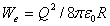Q is the charge in Coulombs and R the radius of the bulb in meters.

When charge Q disappears the tension in the ether, the electric field around Q, will recover. In a shock wave, with the speed of light, the dielectric charge displacement eases around Q. The energy of the electric field discharges means of a magnetic current. The restoration of the balance in the ether is not for real, because the energyWe, present in the ether, has not disappeared. The energy of the field is transformed to electromagnetic energy. The discharge concentrates at the point where charge Q is supposed to be. The charge has disappeared, but in the ether there is still a point-volume. The released energy will cause an electromagnetic oscillation.

To make this process plausible we presume a point-volume where the negative and positive volume (charge) is separated. We start with assuming the positive charge is on the outside of the point-volume (situation 1 in figure 13). The electrostatic force in the point-volume will force both negative and positive charge to move. When situation 2 in figure 13 is reached, there is no separation of charge anymore. The potential energy has become pure magnetic energy; the movement of the negative and/or positive charge presents magnetic energy.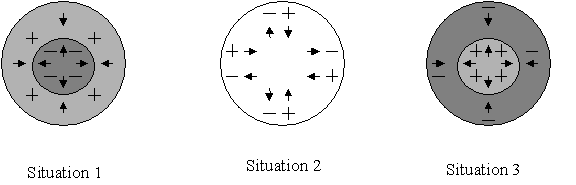Figure 13.      Electromagnetic oscillation in the point-volume.

The oscillation will not stop. The current will proceed and therefore the reversed situation, where the negative charge is situated at the outside of the point-volume, will occur (situation 3).

After situation 3, situation 4 reversed comparable to situation 2 will occur and after that situation 1 will occur again etc. The electric energy of the field has now become an electromagnetic oscillation where electrostatic energy and magnetic energy alternate.

A point-volume in the ether is not alone. The separation in situation 1 will force surrounding point-volumes to separate as well and so on. The ether around the point-volume where Q was situated will oscillate. The influence of the oscillation to the surrounding ether will be limited the oscillation frequency in the central point-volume and the lag of the ether limited  c. The wavelength of the oscillation in the ether is limited. The purpose of the above illustration is to show how it is possible that an electromagnetic oscillation occurs in the ether.

Apart from the described oscillation one can also imagine oscillations in point-volumes that are not in balance with each other. When point-volumes are oscillating independently, not in phase with each other like in the described oscillation, then one can speak of chaotic oscillation. When point-volumes are oscillating independently of each other the interaction between point-volumes will be chaotic. This form of oscillation has the characteristics of thermal radiation.

The chaotic oscillation of the ether, the interaction of all point-volumes not oscillating in phase, is transferred to particles in the ether. Nucleus, electrons and molecules placed in the ether will gain chaotic oscillation when the chaos of their oscillation is less than the chaos in the surrounding ether. The opposite will occur when the temperature of matter, the chaos of oscillation, is higher than the surrounding ether and the matter will give up energy to the ether diminishing the own chaotic oscillation through enlarging the oscillation of the surrounding ether. Only when the temperature is zero degrees Kelvin there is no chaotic vibration in ether and matter.

This approach offers a different point of view towards the background radiation observed in space surrounding the earth. The background radiation, in all directions, agrees with a temperature of approx. 3 degrees Kelvin. This radiation can now be explained as the chaotic oscillation of space around us: the temperature of space. In accordance to this perception the background radiation is the chaotic oscillation energy of ether. The consequence of this is that vacuum may contain (dark) energy and therefore (dark) mass.

The above arguments have no consequence. The illustration shows only how the ether might handle energy in different forms, consistent with the physical properties of a vacuum. Proof that the ideas are valid is not obtained. It only indicates that it might be so. The ether presented can be responsible for the observed physical phenomena.

Until now the ether is described means of the influence of a particle with charge Q. Because electron and proton are stable we had to assume that the positive (+Qe) and negative (-Qe) charge of point-volumes surrounding particle Q cannot leave the neutral point-volume freely. Would it be possible for the charge or a fraction thereof to leave the point-volume and penetrate the charge, then charged particles would not be stable under the sketched circumstances.

The ether, the point-volume, as described needs to have one more characteristic to be able to explain stable particles like electrons, protons and neutrons. Apart from the earlier assumed possible charge separation in the point-volume, the positive and negative charge must also be able to leave the point-volume under restricted circumstances.

We first had to assume that the negative and or positive charges cannot be free to leave the point-volume because then the charge in the point-volumes surrounding the charged particle Q would penetrate the charged particle and destroy respectively annihilate it.

When we however assume that the positive or negative charge can (partly) leave the point-volume and penetrate in other point-volumes, the stable particles like electron and proton must have characteristics that prevent the charge of the point-volumes to penetrate and annihilate these particles.

A stable particle has to have an entity that prohibits the charge in ether to penetrate the particle when we assume the charge can leave the point-volumes.

Next chapter: The electron